#The Polar Form of a Complex Number# The unit circle

The fundamental trigonometric identity (i.e the Pythagorean theorem) is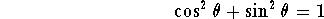From this we can see that the complex numbers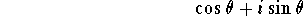are points on the circle of radius one centered at the origin.

Think of the point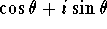moving counterclockwise around the circle as the real numbermoves from left to right. Similarly, the point moves clockwise ifdecreases. And whetherincreases or decreases, the point returns to the same position on the circle wheneverchanges by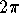or by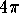or by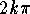where k is any integer.

Exercise: Verify that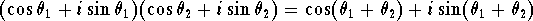Exercise: Prove de Moivre's formula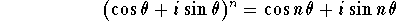Now picture a fixed complex number on the unit circle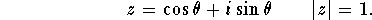Consider multiples of z by a real, positive number r.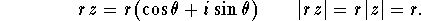As r grows from 1, our point moves out along the ray whose tail is at the origin and which passes through the point z. As r shrinks from 1 toward zero, our point moves inward along the same ray toward the origin. The modulus of the point is r. We call the anglewhich this ray makes with the x-axis, the argument of the number z. All the numbers rz have the same argument. We write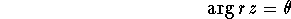Just as a point in the plane is completely determined by its polar coordinates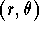, a complex number is completely determined by its modulus and its argument.

Notice that the argument is not defined when r=0 and in any case is only determined up to an integer multiple of.

We now have a geometric interpretation of multiplication![Algebra] [Complex Variables]
[Geometry] [Trigonometry ]
[Calculus] [Differential Equations] [Matrix Algebra]S.O.S MATHematics home page

Do you need more help? Please post your question on our S.O.S. Mathematics CyberBoard.Author: Michael O'Neill